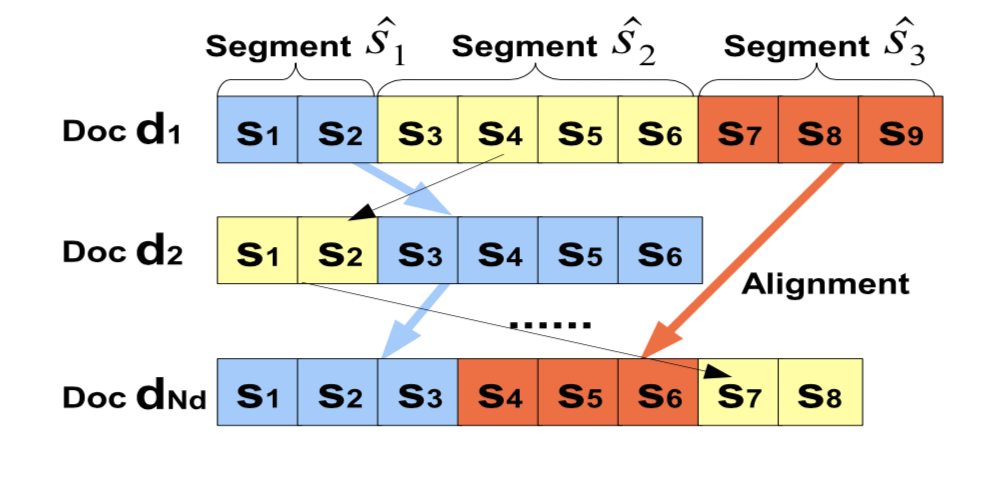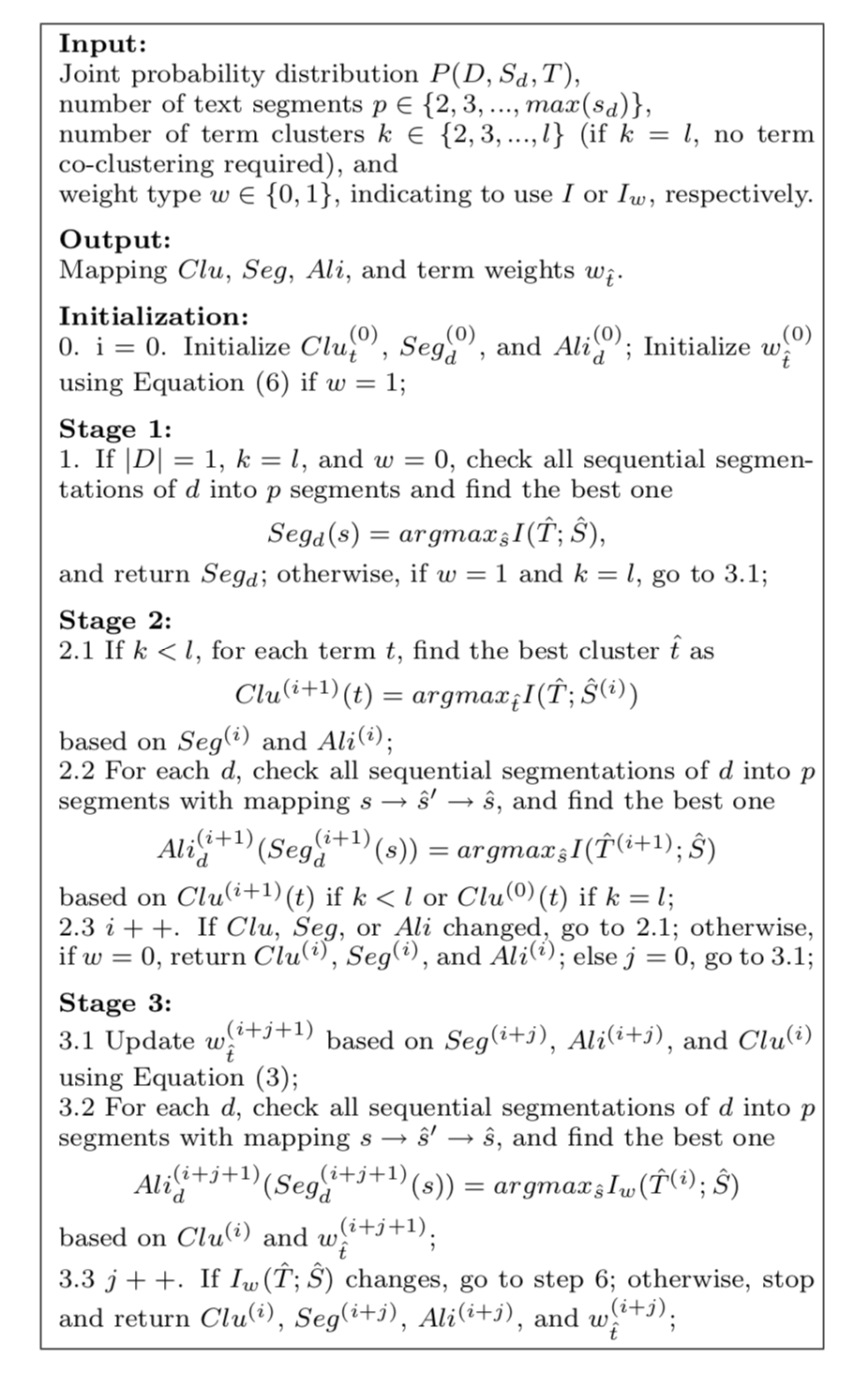terms集合 $T=\lbrace t_1, t_2, …, t_l\rbrace$
documents集合 $D=\lbrace d_1, d_2, …, d_n\rbrace$

Segments集合（准确的说是topic集合，这里的每个segment不是各个文档的片段，而是一个全局topic）$\widehat{S}=\lbrace \widehat{s_1}, \widehat{s_2}, …, \widehat{s_p}\rbrace$ ,也就是每个文档的$\widehat{s_1}$的topic都是相同的，尽管有些documents的$\widehat{s_1}$是空的，也就是不存在对应的sentence。也就是正如前面所说的，论文中这里指的segment其实就是topic（比较迷惑），而topic数目是要预定义的（尽管这个数字一般是没法确定，取一个大的数字是比较合适的，但是增加计算复杂度。）

\begin{aligned} Seg_d : \lbrace s_1, s_2, ..., s_{n_d}\rbrace \to \lbrace \widehat{s_{d1}}, \widehat{s_{d2}}, ..., \widehat{s_{?}}\rbrace \end{aligned}

$\lbrace \widehat{s_{d1}}, \widehat{s_{d2}}, ..., \widehat{s_{?}}\rbrace$ 表示这个文档的segmentation，其中每个$\widehat{s}$表示文档的片段。 以及各个文档的segmentation的alignment，也就是对于每个文档： \begin{aligned} Ali_d=\lbrace \widehat{s_{d1}}, \widehat{s_{d2}}, ..., \widehat{s_{?}}\rbrace \to \lbrace \widehat{s_1}, \widehat{s_2}, ..., \widehat{s_p}\rbrace \end{aligned}

(这里原文的表述和公式相当让人迷惑，所以做了调整)

\begin{aligned} I(X;Y) = \sum_{x\in X}\sum_{y\in Y}p(x, y)log\frac{p(x,y)}{p(x)p(y)} \end{aligned}

\begin{aligned} I(\widehat{T};\widehat{S}) = \sum_{\widehat{t}\in \widehat{T}}\sum_{\widehat{s}\in \widehat{S}}p(\widehat{t}, \widehat{s})log\frac{p(\widehat{t},\widehat{s})}{p(\widehat{t})p(\widehat{s})} \end{aligned}